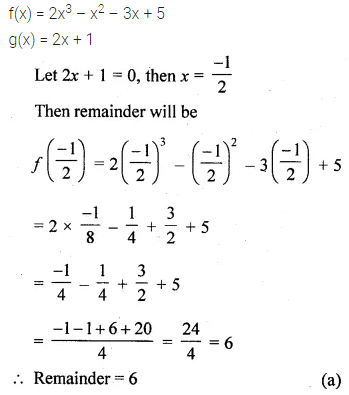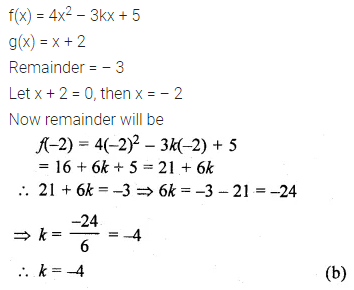# ML Aggarwal Class 10 Solutions for ICSE Maths Chapter 6 Factorization MCQS

## ML Aggarwal Class 10 Solutions for ICSE Maths Chapter 6 Factorization MCQS

ML Aggarwal Class 10 Solutions for ICSE Maths Chapter 6 Factorization MCQS

Choose the correct answer from the given four options (1 to 5):

Question 1.
When x3 – 3x2 + 5x – 7 is divided by x – 2,then the remainder is
(a) 0
(b) 1
(c) 2
(d) -1
Solution:Question 2.
When 2x3 – x2 – 3x + 5 is divided by 2x + 1, then the remainder is
(a) 6
(b) -6
(c) -3
(d) 0
Solution:Question 3.
If on dividing 4x2 – 3kx + 5 by x + 2, the remainder is -3 then the value of k is
(a) 4
(b) -4
(c) 3
(d) -3
Solution:Question 4.
If on dividing 2x3 + 6x2 – (2k – 7)x + 5 by x + 3, the remainder is k – 1 then the value of k is
(a) 2
(b) -2
(c) -3
(d) 3
Solution:Question 5.
If x + 1 is a factor of 3x3 + kx2 + 7x + 4, then the value of k is
(a) -1
(b) 0
(c) 6
(d) 10
Solution:ML Aggarwal Class 10 Solutions for ICSE Maths